# Machine Learning — Latent Dirichlet Allocation LDA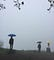Guess where is it?

Public opinion dominates election results. Unfortunately, with the proliferation of social media, public opinion can be manipulated by technology. For example, a policy writer of a candidate can collect billions of tweets (and posts) to analyze voter sentiments. Instead of pre-categorized the topics, the policy writer wants the tweets to self-identify what are the important topics that voters care.

LDA is usually one of the last topics taught in an ML class. Maybe, it is too close to the holiday break, the topic is usually covered in a breeze. In reality, it is important but involves so many concepts that it is hard to cover in a short time. In this article, we will dig deeper into those theories and the math involved.

None of the tweets will explicitly say what topics they are. In fact, a single tweet may be related to multiple topics and a single word may imply different topics. Our objective is grouping words in the tweets into K topics (say 4) and rates the likelihood of each word for each topic. But remember that a word may belong to multiple topics. From the ML perspective, we group words based on the criteria that they should frequently appear in the same tweet. After the grouping, we review words that belong to the same group. From there, we may realize what the topic is.

This trained model can be repurposed as a generative model that generates a document by itself. Say, we have a joint probability model for the topic proportions of a document. For example, p(T₁=0.7, T₂=0.3, T₃=0, T₄=0) indicates the chance that a document has 70% of words related to topic T₁ and 30% of words related to topic T₂.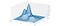Demonstrate as p(T₁, T₂) for simplicity

And, for each topic, we have a list of words with its occurrence likelihood. However, we will not include un-important words, like those stop words “a”, “the”, “and” etc…, in the list

To generate a new document, we sample from p to get T₁’, T₂’, T₃’, T₄’. These values add up to one and represent the ratio of the topics that the new document should cover. For each word in the document, we sample a topic Tc using the probability value from T₁’, T₂’, T₃’, T₄’. Then, we choose a word according to the occurrence likelihood in the chosen topic Tc.

The generated document is unlikely grammatically sound. But throw in the language model and more complex models in selecting words based on previous selections, we may not be too far from replacing a human policy writer from a machine. Indeed, the state of the art technology in the generative model produces very realistic articles.

Let’s get into a few more examples. Here is a visualization on the possible topics for the Star Wars Wikipedia page. To my surprise, Star Wars is about politics as much as entertainment.

Here is another analysis which it finds the frequent topics that a Wikipedia page relates to.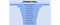Source

The second example below models the topics on the NY Times articles. The following are the 10 topics that it discovered with the top 10 words associated. If we examine them closely, it will not be hard to discover what these topics are.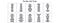Source

Topic modeling is mainly unsupervised learning that categorizes, organizes, tags and generates information. So given a corpus, we want to find the distribution of words for each topic. But we are dealing with a chicken and egg problem. We don’t know the topics a document may cover. But we expect them to cover a small set of topics and each topic should have the smallest possible set of words. But these objectives can be contradictive. When parsing all the documents, we may find a cluster of words in a set of documents to identify a potential topic. But how do we identify this set of documents? There are too many moving parts. Changing one will change the other. We need an algorithm that can solve this dilemma. Let’s frame this topic modeling technically.

Each topic is simply a distribution over words. Each document contains a mixture of topics and words are drawn from these topics.

# Latent Dirichlet allocation (LDA)

Let’s imagine how a generative model produces an article discussed before. But first, let’s talk about the Dirichlet distribution. We don’t need to dig too deep in the math but it will be nice to know what it does. Dirichlet distribution is defined as: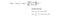Dirichlet distribution definition

where Τ is the gamma function. For a joint probability with m variables, the output of Dirichlet is m-dimensional and takes m parameters to model it. For example, the model p(x₁, x₂, x₃, x₄) will have model parameters α₁, α₂, α₃, and α₄. In LDA, we model both word distribution for each topic and topic proportion for each document using Dirichlet distributions. For example, by learning αᵢ, we learn the topics proportion that a document may cover. The higher the value for αᵢ relative to others, the more likely the topic i will be picked.

In a Dirichlet distribution, the sampled value from p is a probability distribution (e.g. x₁=0.7, x₂=0.2, x₃=0.1, and x₄=0) which always sums up to one. That is why we call Dirichlet distribution a distribution of distributions. There is a special case in the Dirichlet distribution called symmetric Dirichlet distribution. All αᵢ will be equal and therefore, we use a single scalar α in representing the model. As the value of α decreases, the sparsity increases, i.e. most values in a sampled data will be zero or close to zero (e.g. x₁=0.9, x₂=0, x₃=0.1, and x₄=0).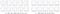Modified from source

This sparsity can be parameterized in any Dirichlet distribution, not just the symmetric Dirichlet distribution. It allows us to control the sparsity of topics proportions or word distributions. For example, it allows us to build a model that a document should cover a small number of topics only (high sparsity).

Below are the steps in generating a document. In step 1, we have a V-dimensional Dirichlet distribution for the word distribution for each topic (βk). V is the size of the vocabulary and we have K topics. In step 2(a), we draw a topic proportions for each document. In step 2(b), we sample a topic assignment for each word and we draw a word from the word distribution for the corresponding topic.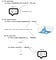Algorithm skeleton is originated from here

Like other ML modelings, we want to infer the joint probability given our observations,

We infer the hidden variables or latent factors θ, z, β by observing the corpse of documents, i.e. finding p(θ, z, β | w).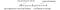Modified from source

But before submerging ourselves into equations and models, let’s have a big picture of what we are doing. For each document, we keep track of the topic proportions θ and the most likely topic assignment z for each document word. In step 3 to 8, we fix the word distribution β for each topic. We find what is the most likely topic proportion θ and z for each document word.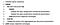Source

Then in step 9, we turn the table around and aggregate the results from all documents to update the word distribution β for each topic. Since θ, β and z are interdependent, we cannot optimize them all at once. Therefore we optimize one variable at a time while holding the other variables fixed. That is the alternating optimizing step of 5, 6, and 9 above. Even this may lead to a local optimal or a suboptimal solution, the generated solutions are often high in quality.

However in LDA, θ, β and z will be modeled with probability models instead of a point estimate which only hold the most likely estimate only. These models keep track of all likelihood and its certainty. So we keep richer information in each iteration. We can visualize LDA as 64-bit double-precision floating-point operations while a point estimate truncates all the values into integers for every iteration. Technically, the probability model makes training easier and more stable. But this makes the math very hard.

Let’s use a Graphical model to demonstrate the variable dependency. For example, we link Z (the topic selection) and β (the word distribution of a topic) to W (topic selection of the word) to show W depends on Z and β.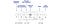Modified from source

(The equations are originated from different sources. Even they may come from the same author, slightly different notations or indexing are used. Be aware.)

The joint probability modeled by the Graphical model is:

• βk is the word distribution for the topic k.
• θ is the topic proportions of a document (e.g. 70% climate, 30% economy).
• z is the topic assignment of a word in a document.

Topic parameter η and proportions parameter α are treated as some prior knowledge on the word distribution and the topic proportion distribution respectively. There are different approaches to model or choose η and α. But for our discussion, we stick with a common approach in treating them as hyperparameters that can be chosen heuristically. The topic parameter η discussed here is a scalar parameter for the Dirichlet distribution which influences the sparsity of the Dirichlet distribution. For the proportions parameter α, we will use a vector. From some perspective, these parameters allow a human expert to influence the Dirichlet distribution (using expert knowledge or empirical experiment) rather than learning it purely from the data. These parameters act as prior to the posterior calculation.

Using this joint probability, we infer the distribution of the hidden variables (β, θ, z) given the evidence w.

However, inferring a posterior is hard in general. Integrating over variables β, θ is intractable. They depend on each other and it is NP-hard.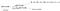Modified from source

So we are going to approximate the posterior p(β, θ, z|w) with distribution q(β, θ, z) using variance inference. The key concept of variance inference is approximate p with q using some known families of distribution that is easy to model and to analyze.

Then, we train the model parameters to minimize the KL-divergence between q and p.

Mean-field variational inference

However, it remains extremely hard to model q for a high dimensional distribution. To reduce the complexity further, we make bold independence assumptions similar to the Graphical model and break down the joint probability into independent subcomponents.Source

Here is a more detail description for each sub-components.

Here, instead of modeling a joint probability for multiple variables, we model each variable independently, i.e. each variable vᵢ will be model as qᵢ(vᵢ|ρᵢ) with a specific distribution family that found appropriate, e.g. using a multinomial distribution for the topic assignment.

This is called the Mean-field variational inference which breaks up the joint distribution into distributions of individual variables that are tractable and easy to analyze.

In real life, many independence assumptions may be false. But we should view it as imperfect rather than wrong. Indeed, empirical results often demonstrate good quality results.

As mentioned before, to optimize inter-dependent variables, we separate them into groups with variables independent of each other in each group. In LDA, we have the parameters modeling the topic assignment and the topic proportions separated.Source

The most difficult steps are step 5,6 and 9. So, let’s see how we model p with q by minimizing their KL-divergence. As shown in a previous article, it is not easy to optimize KL-divergence directly. So let us introduce the Evidence lower bound (ELBO) below:

Let’s evaluate the relationship between the KL-divergence and ELBO.

Since KL-divergence is always positive, log Z is always greater or equal ELBO. ELBO is the lower bound of the log evidence (log Z) for any q. However, when q equals p, log Z and ELBO have the same value, i.e. by maximizing ELBO, we are minimizing KL-divergence.

When minimizing ELBO, we don’t need Z. No normalization is needed. In contrast, KL’s calculation needs the calculated entity to be a probability distribution. Therefore, we need to compute the normalization factor Z if it is not equal to one. Calculating Z is hard. This is why we calculate ELBO instead of KL-divergence.

The corresponding ELBO that we want to maximize in LDA is:Source

where the expectation value is calculated w.r.t. q.

Graphical model

Let’s simplify the Graphical model so we can explain the LDA in steps.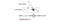Source

where xᵢ is an observation depending on β and zᵢ. We want to model the posterior underlined in red by factorizing p into sub-components. We will model these conditional probabilities asSource

R.H.S. is the exponential family of distribution.

It is a generalization of many distributions including Gaussian, binomial, multinomial, Poisson, Gamma, Dirichlet and beta distributions (details). This makes the equations a little bit abstract but establishes a general framework for us to move forward. Also, it provides a general solution for some problems that thought to be tough to solve. Let’s demonstrate how to express a Dirichlet distribution as an exponential family of distribution.

So instead of modeling the Dirichlet distribution with α, it is generalized to the exponential family modeled with η. For each type of distribution, we will have a specific definition for T(θᵢ) and h(x). In a Dirichlet distribution, T(θᵢ) = log θᵢ and h(x)=1. So if the Dirichlet distribution has α = (2, 2, 2), the new model parameter for the exponential family of distribution will be η = (1, 1, 1).

Here are the details of expressing Dirichlet distribution and the multinomial distribution into the exponential family. It looks scary but should be manageable. But again, we don’t need to over-worry this detail too much.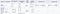Modified from source

ELBO & Mean-field variational inference

Next, we apply mean-field variation inference to approximate p with q. To minimize the KL-divergence, we maximize the ELBO L in finding a variation distribution q(β, z) that approximate the posterior p(β, z|x).

q will be approximate with the mean-field variation inference.

The corresponding model will be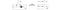Source

with β and zmodeled as

Our objective is to approximate p with q by optimizing the ELBO below (R.H.S.) w.r.t. λ and 𝜙ᵢ.

We take a derivative on L w.r.t. λ and set it to 0, the optimal λ* will be

And the optimal 𝜙ᵢ* will be

(Don’t worry about the proof and the equations. Some details will be covered in the next section.)

Usually, the expectation E[f(x, y)] is defined as:

But in our context, we calculate the expectation over all variables except the one we want to optimize (say 𝜙, λ in LDA or x below).

Again, we optimize them in alternating steps.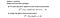Source

Now, let’s extend the graphical model to resemble the LDA problem that we discuss before.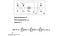Modified from source

The topic proportions θ will be modeled by a Dirichlet distribution with parameter γ. The topic assignment Z, say [trade], will be modeled by Multinomial distribution with 𝜙.

For each document, the optimal 𝜙 and γ in each iteration will be.

Once the documents are processed, we update the parameters for the topics.

where the summation indicates the expectation that the word w is assigned to topic k. In a Dirichlet distribution, as λ_kw increases, the chance of selecting the word w in topic k also increases.

Here are steps in performing one iteration of the Mean-field variational inference. In step 2(b), we evaluate the expectation 𝔼[log θ] and 𝔼[log β] into digamma function (details later).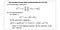Modified from source

where the digamma function is

# Expectation

In variational inference, the optimal parameter is often expressed in some expectation form.

We usually decompose η into simpler components and compute the corresponding expectation. One of the common expectation we want to compute is E[log θ]. Let’s see how it is computed if θ is Dirichlet distributed.

First, we transform the Dirichlet distribution p(θ|α) into the exponential family of distribution.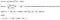Modified from source

Next, we will take advantage of a well-known property for the exponential family of distribution. Its first derivative of A(η) equals the expectation of sufficient statistics. For T(θᵢ) =log θᵢ, A’(η) = 𝔼(log (θ|α)). So the expected value we want to calculate equals the derivative of A(η). With A(η) equals

The expected log, 𝔼(log (θ|α)), will be:

We will use this in the LDA proof.

# LDA Proof

Now, let’s prove the solution for the LDA that we skip before and fill up some of the details in this section. The proof here is originated from the LDA paper with a slightly different notation. So let’s align with the notation first. Here is the factorization of the joint distribution.

And the Graphical model is: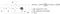Modified from source

The ELBO L is:

With the concept in Mean-field variational inference, q is factorized as:

We expand the first term in ELBO according to the Graphical model. For the second term, we apply the assumption that the joint probability of q can be broken up into individual variables. Here is the expanded result.

Next, we optimize L w.r.t. γ and 𝜙. Expanding all five terms above in the R.H.S. takes times. So we will only demonstrate the expansion for the first term of L (log p(θᵢ|α)) in our discussion.

Recall θ is Dirichlet distributed with parameter α.

In the last section, if θ is Dirichlet distributed, we also establish

So the first term E[log p(θ|α)] equals

The ELBO can be further expanded below with each term in a separate line.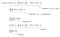Modified from source

where Ψ is

Next, let’s optimize L relative to the variational parameters 𝜙 and γ.

Optimize 𝜙 modeled as a multinomial distribution

𝜙ni is the probability that the nth word in a doc is in the topic i. 𝜙 has a multinomial distribution. That is 𝜙ni sums to one over all the topics. Remove terms that are un-related with 𝜙 from L. We optimize the reduced L w.r.t. 𝜙. But to enforce the constraint, we add a Lagrange multiplier below.

By setting its derivative to 0, we get the optimal value for 𝜙ni.

Optimize γ modeled as a Dirichlet distribution

Now, it is time to optimize the topic proportions for L w.r.t γ. γ models the topic proportions with a Dirichlet distribution.

As indicated by the summation, it is natural to see γ increases as more words in the document belonging to the topic i. As γis larger than other topics, the corresponding topic will have a higher chance to be picked according to the Dirichlet distribution which has an expected value of θᵢ calculated as:

Here is the final algorithm after putting everything together.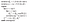Source

# Dirichlet Distribution & Multinomial

Dirichlet distribution is the prior conjugate of the multinomial distribution. In Bayes’ Theorem, if the prior is Dirichlet distributed and the likelihood is multinomial distributed, the posterior will be Dirichlet distributed and can be computed easily (detail).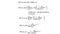Modified from source

That is some of the math behind LDA that makes life easier.

# Topic models

As mentioned in the beginning, our model will not provide a grammatically correct article or generate words with coherent meaning. One major problem is our graphical model is too simple. Words are sampled independently.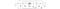Source

In this section, we will briefly cover other possibilities in the Graphical model to expand its application.

Correlated topic models

By drawing components from a Gaussian distribution, the value xᵢ in a sample data will be correlated by the covariance matrix Σ. For example, topics in sequential words can be correlated now.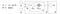Modified from source

Dynamic topic models

Topics of interests may change over time.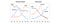Source

Even we can collect billions of tweets, we may divide it chronically to identify the shift of public interests. For example, βkt will be the word distribution for topic k at time t. We can then model the dependency between consecutive time periods.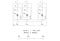Modified from source

# More thoughts

This article is long but I hope you understand LDA much deeper in theory and in math now.

# Credits and references

Latent Dirichlet Allocation paper

Topic models

Probabilistic topic models

Welcome to a place where words matter. On Medium, smart voices and original ideas take center stage - with no ads in sight. Watch
Follow all the topics you care about, and we’ll deliver the best stories for you to your homepage and inbox. Explore
Get unlimited access to the best stories on Medium — and support writers while you’re at it. Just \$5/month. Upgrade